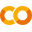# MNIST with SciKit-Learn and skorch¶

This notebooks shows how to define and train a simple Neural-Network with PyTorch and use it via skorch with SciKit-Learn.Run in Google ColabView source on GitHub

Note: If you are running this in a colab notebook, we recommend you enable a free GPU by going:

Runtime   →   Change runtime type   →   Hardware Accelerator: GPU

If you are running in colab, you should install the dependencies and download the dataset by running the following cell:

In :
! [ ! -z "\$COLAB_GPU" ] && pip install torch scikit-learn==0.20.* skorch

In :
from sklearn.datasets import fetch_openml
from sklearn.model_selection import train_test_split
import numpy as np


## Loading Data¶

Using SciKit-Learns fetch_openml to load MNIST data.

In :
mnist = fetch_openml('mnist_784', cache=False)

In :
mnist.data.shape

Out:
(70000, 784)

## Preprocessing Data¶

Each image of the MNIST dataset is encoded in a 784 dimensional vector, representing a 28 x 28 pixel image. Each pixel has a value between 0 and 255, corresponding to the grey-value of a pixel.
The above featch_mldata method to load MNIST returns data and target as uint8 which we convert to float32 and int64 respectively.

In :
X = mnist.data.astype('float32')
y = mnist.target.astype('int64')


As we will use ReLU as activation in combination with softmax over the output layer, we need to scale X down. An often use range is [0, 1].

In :
X /= 255.0

In :
X.min(), X.max()

Out:
(0.0, 1.0)

Note: data is not normalized.

In :
X_train, X_test, y_train, y_test = train_test_split(X, y, test_size=0.25, random_state=42)

In :
assert(X_train.shape + X_test.shape == mnist.data.shape)

In :
X_train.shape, y_train.shape

Out:
((52500, 784), (52500,))

## Build Neural Network with Torch¶

Simple, fully connected neural network with one hidden layer. Input layer has 784 dimensions (28x28), hidden layer has 98 (= 784 / 8) and output layer 10 neurons, representing digits 0 - 9.

In :
import torch
from torch import nn
import torch.nn.functional as F

In :
torch.manual_seed(0);
device = 'cuda' if torch.cuda.is_available() else 'cpu'

In :
mnist_dim = X.shape
hidden_dim = int(mnist_dim/8)
output_dim = len(np.unique(mnist.target))

In :
mnist_dim, hidden_dim, output_dim

Out:
(784, 98, 10)

A Neural network in PyTorch's framework.

In :
class ClassifierModule(nn.Module):
def __init__(
self,
input_dim=mnist_dim,
hidden_dim=hidden_dim,
output_dim=output_dim,
dropout=0.5,
):
super(ClassifierModule, self).__init__()
self.dropout = nn.Dropout(dropout)

self.hidden = nn.Linear(input_dim, hidden_dim)
self.output = nn.Linear(hidden_dim, output_dim)

def forward(self, X, **kwargs):
X = F.relu(self.hidden(X))
X = self.dropout(X)
X = F.softmax(self.output(X), dim=-1)
return X


Skorch allows to use PyTorch's networks in the SciKit-Learn setting.

In :
from skorch import NeuralNetClassifier

In :
net = NeuralNetClassifier(
ClassifierModule,
max_epochs=20,
lr=0.1,
device=device,
)

In :
net.fit(X_train, y_train);

  epoch    train_loss    valid_acc    valid_loss     dur
-------  ------------  -----------  ------------  ------
1        0.8321       0.8828        0.4077  0.7626
2        0.4306       0.9110        0.3121  0.4984
3        0.3623       0.9221        0.2649  0.5147
4        0.3241       0.9298        0.2457  0.5040
5        0.2942       0.9373        0.2129  0.5629
6        0.2707       0.9411        0.1974  0.5093
7        0.2554       0.9439        0.1836  0.5055
8        0.2487       0.9480        0.1754  0.5102
9        0.2276       0.9473        0.1730  0.5055
10        0.2229       0.9524        0.1612  0.4966
11        0.2158       0.9511        0.1600  0.5048
12        0.2059       0.9556        0.1501  0.4979
13        0.1988       0.9572        0.1429  0.4973
14        0.1934       0.9563        0.1460  0.4981
15        0.1915       0.9595        0.1355  0.5030
16        0.1881       0.9607        0.1325  0.5013
17        0.1816       0.9602        0.1302  0.5003
18        0.1796       0.9601        0.1285  0.4977
19        0.1767       0.9624        0.1248  0.5056
20        0.1716       0.9628        0.1236  0.5080


## Prediction¶

In :
predicted = net.predict(X_test)

In :
np.mean(predicted == y_test)

Out:
0.962

An accuracy of nearly 96% for a network with only one hidden layer is not too bad

# Convolutional Network¶

PyTorch expects a 4 dimensional tensor as input for its 2D convolution layer. The dimensions represent:

• Batch size
• Number of channel
• Height
• Width

As initial batch size the number of examples needs to be provided. MNIST data has only one channel. As stated above, each MNIST vector represents a 28x28 pixel image. Hence, the resulting shape for PyTorch tensor needs to be (x, 1, 28, 28).

In :
XCnn = X.reshape(-1, 1, 28, 28)

In :
XCnn.shape

Out:
(70000, 1, 28, 28)
In :
XCnn_train, XCnn_test, y_train, y_test = train_test_split(XCnn, y, test_size=0.25, random_state=42)

In :
XCnn_train.shape, y_train.shape

Out:
((52500, 1, 28, 28), (52500,))
In :
class Cnn(nn.Module):
def __init__(self):
super(Cnn, self).__init__()
self.conv1 = nn.Conv2d(1, 32, kernel_size=3)
self.conv2 = nn.Conv2d(32, 64, kernel_size=3)
self.conv2_drop = nn.Dropout2d()
self.fc1 = nn.Linear(1600, 128) # 1600 = number channels * width * height
self.fc2 = nn.Linear(128, 10)

def forward(self, x):
x = F.relu(F.max_pool2d(self.conv1(x), 2))
x = F.relu(F.max_pool2d(self.conv2_drop(self.conv2(x)), 2))
x = x.view(-1, x.size(1) * x.size(2) * x.size(3)) # flatten over channel, height and width = 1600
x = F.relu(self.fc1(x))
x = F.dropout(x, training=self.training)
x = self.fc2(x)
x = F.softmax(x, dim=-1)
return x

In :
cnn = NeuralNetClassifier(
Cnn,
max_epochs=15,
lr=1,
optimizer=torch.optim.Adadelta,
device=device,
)

In :
cnn.fit(XCnn_train, y_train);

  epoch    train_loss    valid_acc    valid_loss     dur
-------  ------------  -----------  ------------  ------
1        0.4136       0.9711        0.0949  1.7914
2        0.1402       0.9798        0.0636  1.0294
3        0.1129       0.9811        0.0628  1.0192
4        0.0961       0.9851        0.0482  1.0338
5        0.0847       0.9846        0.0517  1.0152
6        0.0772       0.9864        0.0446  1.0351
7        0.0669       0.9871        0.0442  1.0360
8        0.0638       0.9871        0.0426  1.0318
9        0.0612       0.9886        0.0394  1.0215
10        0.0582       0.9882        0.0410  1.0182
11        0.0541       0.9887        0.0367  1.0259
12        0.0513       0.9894        0.0378  1.0252
13        0.0481       0.9898        0.0360  1.0383
14        0.0478       0.9898        0.0362  1.0299
15        0.0466       0.9902        0.0352  1.0203

In :
cnn_pred = cnn.predict(XCnn_test)

In :
np.mean(cnn_pred == y_test)

Out:
0.9891428571428571

An accuracy of 99.1% should suffice for this example!